Chapter 25 Geometric Optics

# 201 25.4 Total Internal Reflection

### Summary

• Explain the phenomenon of total internal reflection.
• Describe the workings and uses of fiber optics.
• Analyze the reason for the sparkle of diamonds.

A good-quality mirror may reflect more than 90% of the light that falls on it, absorbing the rest. But it would be useful to have a mirror that reflects all of the light that falls on it. Interestingly, we can produce total reflection using an aspect of refraction.

Consider what happens when a ray of light strikes the surface between two materials, such as is shown in Figure 1(a). Part of the light crosses the boundary and is refracted; the rest is reflected. If, as shown in the figure, the index of refraction for the second medium is less than for the first, the ray bends away from the perpendicular. (Since $\boldsymbol{n_1 > n_2}$, the angle of refraction is greater than the angle of incidence—that is,  $\boldsymbol{\theta _1 > \theta _2}$.) Now imagine what happens as the incident angle is increased. This causes $\boldsymbol{\theta _2}$ to increase also. The largest the angle of refraction $\boldsymbol{\theta _2}$ can be is $\boldsymbol{90 ^{\circ}}$, as shown in Figure 1(b).The critical angle $\boldsymbol{\theta _c}$ for a combination of materials is defined to be the incident angle $\boldsymbol{\theta _1}$ that produces an angle of refraction of $\boldsymbol{90 ^{\circ}}$. That is, $\boldsymbol{\theta _c}$ is the incident angle for which $\boldsymbol{\theta _2 = 90^{\circ}}$. If the incident angle $\boldsymbol{\theta _1}$ is greater than the critical angle, as shown in Figure 1(c), then all of the light is reflected back into medium 1, a condition called total internal reflection.

### Critical Angle

The incident angle $\boldsymbol{\theta _1}$ that produces an angle of refraction of $\boldsymbol{90 ^{\circ}}$ is called the critical angle, $\boldsymbol{\theta _c}$.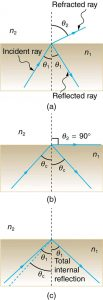Figure 1. (a) A ray of light crosses a boundary where the speed of light increases and the index of refraction decreases. That is, n2 < n1. The ray bends away from the perpendicular. (b) The critical angle θc is the one for which the angle of refraction is . (c) Total internal reflection occurs when the incident angle is greater than the critical angle.

Snell’s law states the relationship between angles and indices of refraction. It is given by

$\boldsymbol{n_1 \;\textbf{sin} \;\theta _1 = n_2 \;\textbf{sin} \;\theta _2}.$

When the incident angle equals the critical angle ($\boldsymbol{\theta _1 = \theta _c}$), the angle of refraction is $\boldsymbol{90^{\circ}}$ ($\boldsymbol{\theta _2 = 90^{\circ}}$). Noting that $\boldsymbol{\textbf{sin} \; 90^{\circ} = 1}$, Snell’s law in this case becomes

$\boldsymbol{n_1 \;\textbf{sin} \;\theta _1 = n_2}.$

The critical angle $\boldsymbol{\theta _c}$ for a given combination of materials is thus

$\boldsymbol{\theta _c = \textbf{sin}^{-1} \;(n_2 / n_1) \;\textbf{for} \; n_1 > n_2}.$

Total internal reflection occurs for any incident angle greater than the critical angle $\boldsymbol{\theta _c}$, and it can only occur when the second medium has an index of refraction less than the first. Note the above equation is written for a light ray that travels in medium 1 and reflects from medium 2, as shown in the figure.

### Example 1: How Big is the Critical Angle Here?

What is the critical angle for light traveling in a polystyrene (a type of plastic) pipe surrounded by air?

Strategy

The index of refraction for polystyrene is found to be 1.49 in Figure 2, and the index of refraction of air can be taken to be 1.00, as before. Thus, the condition that the second medium (air) has an index of refraction less than the first (plastic) is satisfied, and the equation $\boldsymbol{\theta _c = \textbf{sin}^{-1} \;(n_2 / n_1)}$ can be used to find the critical angle $\boldsymbol{\theta _c}$. Here, then, $\boldsymbol{n_2 = 1.00}$ and $\boldsymbol{n_1 = 1.49}$.

Solution

The critical angle is given by

$\boldsymbol{\theta _c = \textbf{sin}^{-1} \; (n_2/n_1)}.$

Substituting the identified values gives

$\boldsymbol{\theta _c = \textbf{sin}^{-1} \; (1.00/1.49) = \textbf{sin}^{-1} \; (0.671) = 42.2^{\circ}}$

Discussion

This means that any ray of light inside the plastic that strikes the surface at an angle greater than $\boldsymbol{42.2^{\circ}}$ will be totally reflected. This will make the inside surface of the clear plastic a perfect mirror for such rays without any need for the silvering used on common mirrors. Different combinations of materials have different critical angles, but any combination with $\boldsymbol{n_1 > n_2}$ can produce total internal reflection. The same calculation as made here shows that the critical angle for a ray going from water to air is $\boldsymbol{48.6^{\circ}}$, while that from diamond to air is $\boldsymbol{24.4^{\circ}}$, and that from flint glass to crown glass is $\boldsymbol{66.3^{\circ}}$. There is no total reflection for rays going in the other direction—for example, from air to water—since the condition that the second medium must have a smaller index of refraction is not satisfied. A number of interesting applications of total internal reflection follow.

# Fiber Optics: Endoscopes to Telephones

Fiber optics is one application of total internal reflection that is in wide use. In communications, it is used to transmit telephone, internet, and cable TV signals. Fiber optics employs the transmission of light down fibers of plastic or glass. Because the fibers are thin, light entering one is likely to strike the inside surface at an angle greater than the critical angle and, thus, be totally reflected (See Figure 2.) The index of refraction outside the fiber must be smaller than inside, a condition that is easily satisfied by coating the outside of the fiber with a material having an appropriate refractive index. In fact, most fibers have a varying refractive index to allow more light to be guided along the fiber through total internal refraction. Rays are reflected around corners as shown, making the fibers into tiny light pipes.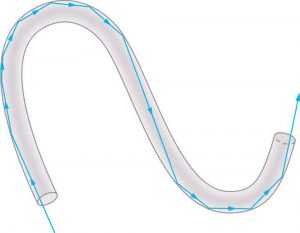Figure 2. Light entering a thin fiber may strike the inside surface at large or grazing angles and is completely reflected if these angles exceed the critical angle. Such rays continue down the fiber, even following it around corners, since the angles of reflection and incidence remain large.

Bundles of fibers can be used to transmit an image without a lens, as illustrated in Figure 3. The output of a device called an endoscope is shown in Figure 3(b). Endoscopes are used to explore the body through various orifices or minor incisions. Light is transmitted down one fiber bundle to illuminate internal parts, and the reflected light is transmitted back out through another to be observed. Surgery can be performed, such as arthroscopic surgery on the knee joint, employing cutting tools attached to and observed with the endoscope. Samples can also be obtained, such as by lassoing an intestinal polyp for external examination.

Fiber optics has revolutionized surgical techniques and observations within the body. There are a host of medical diagnostic and therapeutic uses. The flexibility of the fiber optic bundle allows it to navigate around difficult and small regions in the body, such as the intestines, the heart, blood vessels, and joints. Transmission of an intense laser beam to burn away obstructing plaques in major arteries as well as delivering light to activate chemotherapy drugs are becoming commonplace. Optical fibers have in fact enabled microsurgery and remote surgery where the incisions are small and the surgeon’s fingers do not need to touch the diseased tissue.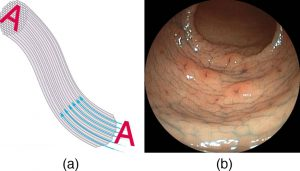Figure 3. (a) An image is transmitted by a bundle of fibers that have fixed neighbors. (b) An endoscope is used to probe the body, both transmitting light to the interior and returning an image such as the one shown. (credit: Med_Chaos, Wikimedia Commons)

Fibers in bundles are surrounded by a cladding material that has a lower index of refraction than the core. (See Figure 4.) The cladding prevents light from being transmitted between fibers in a bundle. Without cladding, light could pass between fibers in contact, since their indices of refraction are identical. Since no light gets into the cladding (there is total internal reflection back into the core), none can be transmitted between clad fibers that are in contact with one another. The cladding prevents light from escaping out of the fiber; instead most of the light is propagated along the length of the fiber, minimizing the loss of signal and ensuring that a quality image is formed at the other end. The cladding and an additional protective layer make optical fibers flexible and durable.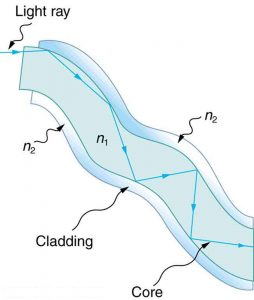Figure 4. Fibers in bundles are clad by a material that has a lower index of refraction than the core to ensure total internal reflection, even when fibers are in contact with one another. This shows a single fiber with its cladding.

### Cladding

The cladding prevents light from being transmitted between fibers in a bundle.

Special tiny lenses that can be attached to the ends of bundles of fibers are being designed and fabricated. Light emerging from a fiber bundle can be focused and a tiny spot can be imaged. In some cases the spot can be scanned, allowing quality imaging of a region inside the body. Special minute optical filters inserted at the end of the fiber bundle have the capacity to image tens of microns below the surface without cutting the surface—non-intrusive diagnostics. This is particularly useful for determining the extent of cancers in the stomach and bowel.

Most telephone conversations and Internet communications are now carried by laser signals along optical fibers. Extensive optical fiber cables have been placed on the ocean floor and underground to enable optical communications. Optical fiber communication systems offer several advantages over electrical (copper) based systems, particularly for long distances. The fibers can be made so transparent that light can travel many kilometers before it becomes dim enough to require amplification—much superior to copper conductors. This property of optical fibers is called low loss. Lasers emit light with characteristics that allow far more conversations in one fiber than are possible with electric signals on a single conductor. This property of optical fibers is called high bandwidth. Optical signals in one fiber do not produce undesirable effects in other adjacent fibers. This property of optical fibers is called reduced crosstalk. We shall explore the unique characteristics of laser radiation in a later chapter.

# Corner Reflectors and Diamonds

A light ray that strikes an object consisting of two mutually perpendicular reflecting surfaces is reflected back exactly parallel to the direction from which it came. This is true whenever the reflecting surfaces are perpendicular, and it is independent of the angle of incidence. Such an object, shown in Figure 5, is called a corner reflector, since the light bounces from its inside corner. Many inexpensive reflector buttons on bicycles, cars, and warning signs have corner reflectors designed to return light in the direction from which it originated. It was more expensive for astronauts to place one on the moon. Laser signals can be bounced from that corner reflector to measure the gradually increasing distance to the moon with great precision.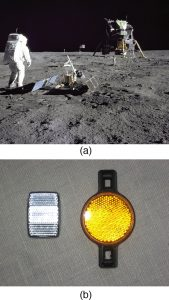Figure 5. (a) Astronauts placed a corner reflector on the moon to measure its gradually increasing orbital distance. (credit: NASA) (b) The bright spots on these bicycle safety reflectors are reflections of the flash of the camera that took this picture on a dark night. (credit: Julo, Wikimedia Commons)

Corner reflectors are perfectly efficient when the conditions for total internal reflection are satisfied. With common materials, it is easy to obtain a critical angle that is less than $\boldsymbol{45^{\circ}}$. One use of these perfect mirrors is in binoculars, as shown in Figure 6. Another use is in periscopes found in submarines.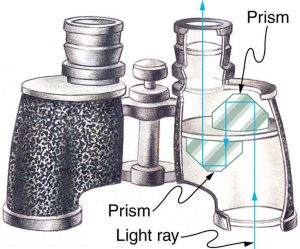Figure 6. These binoculars employ corner reflectors with total internal reflection to get light to the observer’s eyes.

# The Sparkle of Diamonds

Total internal reflection, coupled with a large index of refraction, explains why diamonds sparkle more than other materials. The critical angle for a diamond-to-air surface is only $\boldsymbol{24.4^{\circ}}$, and so when light enters a diamond, it has trouble getting back out. (See Figure 7.) Although light freely enters the diamond, it can exit only if it makes an angle less than $\boldsymbol{24.4^{\circ}}$. Facets on diamonds are specifically intended to make this unlikely, so that the light can exit only in certain places. Good diamonds are very clear, so that the light makes many internal reflections and is concentrated at the few places it can exit—hence the sparkle. (Zircon is a natural gemstone that has an exceptionally large index of refraction, but not as large as diamond, so it is not as highly prized. Cubic zirconia is manufactured and has an even higher index of refraction ($\boldsymbol{\approx 2.17}$), but still less than that of diamond.) The colors you see emerging from a sparkling diamond are not due to the diamond’s color, which is usually nearly colorless. Those colors result from dispersion, the topic of Chapter 25.5 Dispersion: The Rainbow and Prisms. Colored diamonds get their color from structural defects of the crystal lattice and the inclusion of minute quantities of graphite and other materials. The Argyle Mine in Western Australia produces around 90% of the world’s pink, red, champagne, and cognac diamonds, while around 50% of the world’s clear diamonds come from central and southern Africa.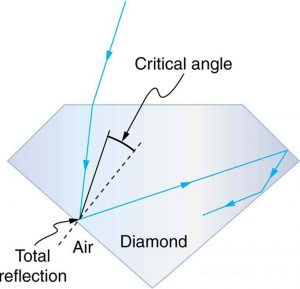Figure 7. Light cannot easily escape a diamond, because its critical angle with air is so small. Most reflections are total, and the facets are placed so that light can exit only in particular ways—thus concentrating the light and making the diamond sparkle.

### PhET Explorations: Bending Light

Explore bending of light between two media with different indices of refraction. See how changing from air to water to glass changes the bending angle. Play with prisms of different shapes and make rainbows.Figure 8. Bending Light

# Section Summary

• The incident angle that produces an angle of refraction of $\boldsymbol{90^{\circ}}$ is called critical angle.
• Total internal reflection is a phenomenon that occurs at the boundary between two mediums, such that if the incident angle in the first medium is greater than the critical angle, then all the light is reflected back into that medium.
• Fiber optics involves the transmission of light down fibers of plastic or glass, applying the principle of total internal reflection.
• Endoscopes are used to explore the body through various orifices or minor incisions, based on the transmission of light through optical fibers.
• Cladding prevents light from being transmitted between fibers in a bundle.
• Diamonds sparkle due to total internal reflection coupled with a large index of refraction.

### Conceptual Questions

1: A ring with a colorless gemstone is dropped into water. The gemstone becomes invisible when submerged. Can it be a diamond? Explain.

2: A high-quality diamond may be quite clear and colorless, transmitting all visible wavelengths with little absorption. Explain how it can sparkle with flashes of brilliant color when illuminated by white light.

3: Is it possible that total internal reflection plays a role in rainbows? Explain in terms of indices of refraction and angles, perhaps referring to Figure 9. Some of us have seen the formation of a double rainbow. Is it physically possible to observe a triple rainbow?Figure 9. Double rainbows are not a very common observance. (credit: InvictusOU812, Flickr)

4: The most common type of mirage is an illusion that light from faraway objects is reflected by a pool of water that is not really there. Mirages are generally observed in deserts, when there is a hot layer of air near the ground. Given that the refractive index of air is lower for air at higher temperatures, explain how mirages can be formed.

### Problems & Exercises

1: Verify that the critical angle for light going from water to air is $\boldsymbol{48.6^{\circ}}$, as discussed at the end of Example 1, regarding the critical angle for light traveling in a polystyrene (a type of plastic) pipe surrounded by air.

2: (a) At the end of Example 1, it was stated that the critical angle for light going from diamond to air is $\boldsymbol{24.4^{\circ}}$. Verify this. (b) What is the critical angle for light going from zircon to air?

3: An optical fiber uses flint glass clad with crown glass. What is the critical angle?

4: At what minimum angle will you get total internal reflection of light traveling in water and reflected from ice?

5: Suppose you are using total internal reflection to make an efficient corner reflector. If there is air outside and the incident angle is $\boldsymbol{45.0^{\circ}}$, what must be the minimum index of refraction of the material from which the reflector is made?

6: You can determine the index of refraction of a substance by determining its critical angle. (a) What is the index of refraction of a substance that has a critical angle of $\boldsymbol{68.4^{\circ}}$ when submerged in water? What is the substance, based on Chapter 25.3 Table 1? (b) What would the critical angle be for this substance in air?

7: A ray of light, emitted beneath the surface of an unknown liquid with air above it, undergoes total internal reflection as shown in Figure 10. What is the index of refraction for the liquid and its likely identification?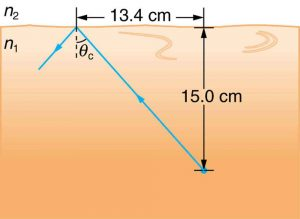Figure 10. A light ray inside a liquid strikes the surface at the critical angle and undergoes total internal reflection.

8: A light ray entering an optical fiber surrounded by air is first refracted and then reflected as shown in Figure 11. Show that if the fiber is made from crown glass, any incident ray will be totally internally reflected.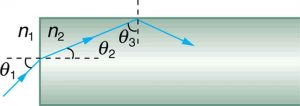Figure 11. A light ray enters the end of a fiber, the surface of which is perpendicular to its sides. Examine the conditions under which it may be totally internally reflected.

## Glossary

critical angle
incident angle that produces an angle of refraction of $\boldsymbol{90^{\circ}}$
fiber optics
transmission of light down fibers of plastic or glass, applying the principle of total internal reflection
corner reflector
an object consisting of two mutually perpendicular reflecting surfaces, so that the light that enters is reflected back exactly parallel to the direction from which it came
zircon
natural gemstone with a large index of refraction

### Solutions

Problems & Exercises

3: $\boldsymbol{66.3^{\circ}}$

5: > 1.414

7: 1.50, benzene

## LicenseCollege Physics: OpenStax by OpenStax is licensed under a Creative Commons Attribution 4.0 International License, except where otherwise noted.